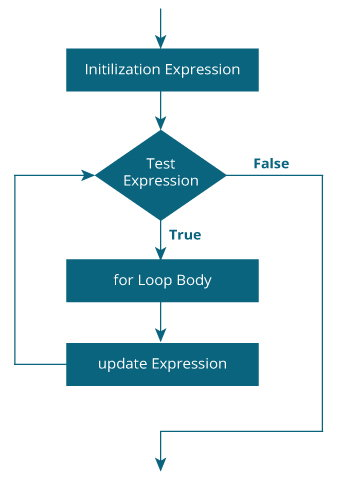# Java for Loop

Loop is used in programming to repeat a specific block of code. In this article, you will learn to create a for loop in Java programming.

Loop is used in programming to repeat a specific block of code until certain condition is met (test expression is `false`).

Loops are what makes computers interesting machines. Imagine you need to print a sentence 50 times on your screen. Well, you can do it by using print statement 50 times (without using loops). How about you need to print a sentence one million times? You need to use loops.

It's just a simple example. You will learn to use `for` loop to write some interesting programs in this article.

## Java for Loop

The syntax of for Loop in Java is:

```for (initialization; testExpression; update)
{
// codes inside for loop's body
}```

### How for loop works?

1. The initialization expression is executed only once.
2. Then, the test expression is evaluated. Here, test expression is a boolean expression.
3. If the test expression is evaluated to `true`,
• Codes inside the body of `for` loop is executed.
• Then the update expression is executed.
• Again, the test expression is evaluated.
• If the test expression is `true`, codes inside the body of `for` loop is executed and update expression is executed.
• This process goes on until the test expression is evaluated to `false`.
4. If the test expression is evaluated to `false`, `for` loop terminates.

### for Loop Flowchart### Example 1: for Loop

``````// Program to print a sentence 10 times

class Loop {
public static void main(String[] args) {

for (int i = 1; i <= 10; ++i) {
System.out.println("Line " + i);
}
}
}
``````

When you run the program, the output will be:

```Line 1
Line 2
Line 3
Line 4
Line 5
Line 6
Line 7
Line 8
Line 9
Line 10```

Here, the variable i is declared and initialized to 1.

Then, the test expression `i <= 10` is evaluated. Since, it's `true`, the body of `for` loop is executed which prints `Line 1` on the screen.

Then, the update expression `++i` is executed. Now, the value of i is increased to 2. Again the expression `i <= 10` is evaluated which is `true` and the body of `for` loop is executed which prints `Line 2` on the screen.

This iteration process goes on until i is 11. When i is 11, expression `i <= 10` is `false` and for loop terminates.

### Example 2: for Loop

``````// Program to find the sum of natural numbers from 1 to 1000.

class Number {
public static void main(String[] args) {

int sum = 0;

for (int i = 1; i <= 1000; ++i) {
sum += i;     // sum = sum + i
}

System.out.println("Sum = " + sum);
}
}
``````

When you run the program, the output will be:

`Sum = 500500`

Here, the variable sum is initialized to 0. Then, in each iteration of for loop, variable sum is assigned `sum + i` and the value of i is increased until i is greater than 1000. For better visualization,

```1st iteration: sum = 0+1 = 1
2nd iteration: sum = 1+2 = 3
3rd iteration: sum = 3+3 = 6
4th iteration: sum = 6+4 = 10
... .. ...

999th iteration: sum = 498501 + 999 = 499500
1000th iteration: sum = 499500 + 1000 = 500500```

### infinite for Loop

If the test expression is never `false`, for loop will run forever. This is called infinite for loop. Let's take an example:

``````// Infinite for Loop

class Infinite {
public static void main(String[] args) {

int sum = 0;

for (int i = 1; i <= 10; --i) {
System.out.println("Hello");
}
}
}``````

Here, the test expression `i <= 10` is never `false` and `hello` is printed infinite number to times (at least in theory).

The initialization, update and test expression used in for statement is optional. Here's an another example of infinite for loop.

``````for ( ; ; ) {

}
``````

### Java for-each Loop

In Java, there is an alternative syntax of `for` loop to work with arrays and collections (known as `for-each` loop).